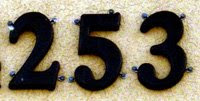## Tuesday, April 28, 2009

### 253

253 = 11 x 23.

253 is a triangular number (the sum of the integers from 1 to 22).

253 has a unique representation as a sum of three squares: 253 = 32 + 102 + 122.

253 is the sum of three positive cubes: 253 = 53 + 43 + 43.

2
5 - 3 is a prime number.253 is a star number. The nth star number is given by the formula 6n(n - 1) + 1.NGC 253, also known as the Silver Coin Galaxy or the Sculptor Galaxy, is a large spiral galaxy in the constellation Sculptor.

Source: Wikipedia